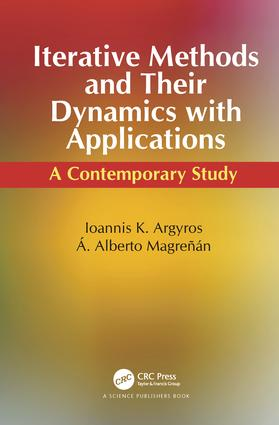# Iterative Methods and Their Dynamics with Applications

## A Contemporary Study, 1st Edition

CRC Press

365 pages | 42 Color Illus.

Hardback: 9781498763608
pub: 2017-02-13
\$175.00
x
eBook (VitalSource) : 9781315153469
pub: 2017-07-12
from \$28.98

FREE Standard Shipping!

### Description

Iterative processes are the tools used to generate sequences approximating solutions of equations describing real life problems. Intended for researchers in computational sciences and as a reference book for advanced computational method in nonlinear analysis, this book is a collection of the recent results on the convergence analysis of numerical algorithms in both finite-dimensional and infinite-dimensional spaces and presents several applications and connections with fixed point theory. It contains an abundant and updated bibliography and provides comparisons between various investigations made in recent years in the field of computational nonlinear analysis.

The book also provides recent advancements in the study of iterative procedures and can be used as a source to obtain the proper method to use in order to solve a problem. The book assumes a basic background in Mathematical Statistics, Linear Algebra and Numerical Analysis and may be used as a self-study reference or as a supplementary text for an advanced course in Biosciences or Applied Sciences. Moreover, the newest techniques used to study the dynamics of iterative methods are described and used in the book and they are compared with the classical ones.

Halley’s method

Introduction

Semilocal convergence of Halley’s method

Numerical examples

Basins of attraction

References

Newton’s method for k-Fréchet differentiable operators

Introduction

Semilocal convergence analysis for Newton’s method

Numerical examples

References

Nonlinear Ill-posed Equations

Introduction

Convergence Analysis

Error Bounds

Numerical Example

References

Sixth-order iterative methods

Introduction

Scheme for constructing sixth-order iterative methods

Sixth-order iterative methods contained in family USS

Numerical Work

Dynamics for method SG

References

Local convergence and basins of attraction of a two-step Newton-like method for equations with solutions of multiplicity greater than one

Introduction

Local convergence

Basins of attraction

Numerical examples

References

Extending the Kantorovich theory for solving equations

Introduction

First convergence improvement

Second convergence improvement

References

Robust convergence for inexact Newton method

Introduction

Standard results on convex functions

Semilocal converngence

Special cases and applications

References

Inexact Gauss-Newton-like method for least square problems

Introduction

Auxiliary Results

Local convergence analysis

Applications and Examples

References

Lavrentiev Regularization Methods for Ill-posed Equations

Introduction

Basic assumptions and some preliminary results

Error Estimates

Numerical Examples

References

King-Werner-type methods of order 1+sqrt(2)

Introduction

Majorizing sequences for King-Werner-type methods (1.3) and (1.4)

Convergence analysis of King-Werner-type methods

Numerical examples

References

Generalized equations and Newton’s method

Introduction

Preliminaries

Semilocal Convergence

References

Newton’s method for generalized equations using restricted domains

Introduction

Preliminaries

Local convergence

Special Cases

References

Secant-like methods

Introduction

Semilocal Convergence analysis of the secant method I

Semilocal Convergence analysis of the secant method II

Local Convergence analysis of the secant method I

Local Convergence analysis of the secant method II

Numerical examples

References

King-Werner-like methods free of derivatives

Introduction

Semilocal convergence

Local convergence

Numerical examples

References

Müller’s method

Convergence ball for method (1.2)

Numerical examples

References

Generalized Newton Method with applications

Introduction

Preliminaries

Semilocal Convergence

References

Newton-secant methods with values in a cone

Introduction

Convergence of the Newton-secant method

References

Gauss-Newton method with applications to convex optimization

Introduction

Gauss-Newton Algorithm and Quasi-Regularity condition

Semilocal convergence for GNA

Specializations and Numerical Examples

References

Directional Newton methods and restricted domains

Introduction

Semilocal convergence analysis

References

Gauss-Newton method for convex optimization

Introduction

Gauss-Newton Algorithm and Quasi-Regularity condition

Semi-local convergence

Numerical Examples

References

Ball Convergence for eighth order method

Introduction

Local convergence analysis

Numerical Examples

References

Expanding Kantorovich’s theorem for solving generalized equations

Introduction

Preliminaries

Semilocal Convergence

References